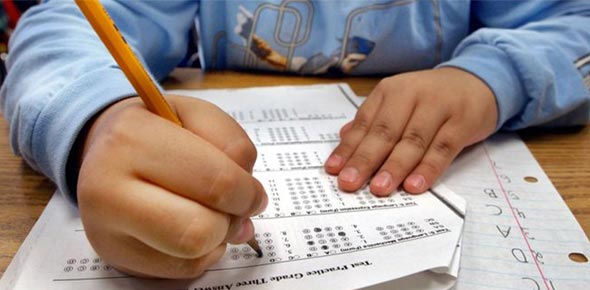# CRCT Grade 2 Math Review (2nd)

10 Questions | Attempts: 954SettingsThis is a CRCT Grade 2 Math review quiz, based upon the sample questions provided by the state of Georgia on their CRCT review site.

• 1.
Look at the number pattern below.26  *  31  *  36  *  ___  *  46  *  51Which number belongs in the blank space?
• A.

35

• B.

40

• C.

41

• 2.
Which number is even?
• A.

23

• B.

20

• C.

29

• D.

25

• 3.
Which is the expanded form of 1,430
• A.

1,000 + 400 + 30

• B.

1000 + 40 + 3

• C.

100 + 40 + 3

• D.

100 + 400 + 3

• 4.
What is the number below? forty-six
• A.

56

• B.

64

• C.

46

• 5.
• A.

\$10

• B.

\$8

• C.

\$6

• D.

\$5

• 6.
Joe wrote down how many times his friends jumped the rope.He put the information in the chart below.Number Of Times Jumped Rope___________________________________Jan                  *  *  *  *  *  *Fred                 *  *  *  *  *Sally                *  *  *  *  *  *  *___________________________________NOTE:  Each * stands for 2 jumps.How many times did Sally jump rope?
• A.

14

• B.

12

• C.

6

• D.

7

• 7.
Look at the number sentence below.3 + 4 = 2 + ____Which number makes the number sentence true?
• A.

7

• B.

9

• C.

5

• D.

1

• 8.
Which number should go in the space?13 + ___ = 17
• A.

5

• B.

30

• C.

4

• D.

21

• 9.
Which is the expanded form of 23,405
• A.

20,000 + 3,000 + 40 + 5

• B.

20,000 + 3,000 + 400 + 5

• C.

2,000 + 300 + 40 + 5

• D.

200 + 300 + 400 + 5

• 10.
Miss Claire's class had 7 gold coins. Mary had won 1 gold coin. Josiah had won 3 gold coins.How many coins did the rest of the class win?
• A.

5

• B.

3

• C.

4

• D.

11

## Related TopicsBack to top
×

Wait!
Here's an interesting quiz for you.